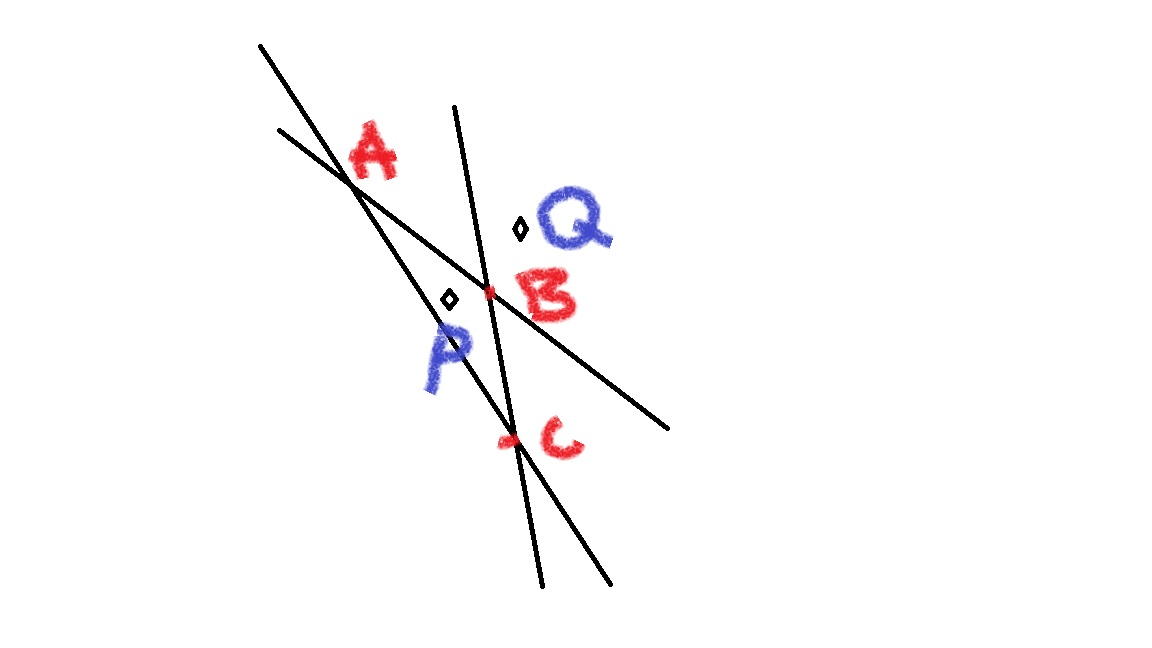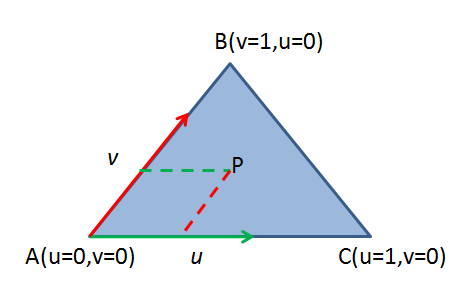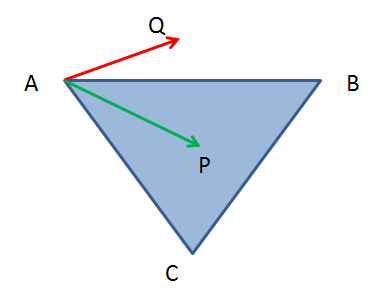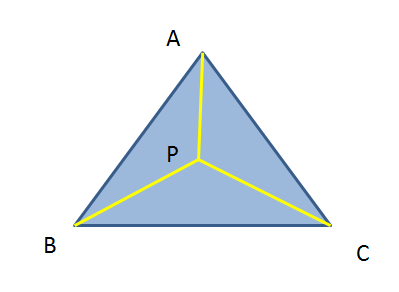• 判断点是否在三角形内
2021-03-04 04:34:45

public class Sanjiao{

public static void main(String[]args){

Triangle p = newTriangle();

if(p.Sanjiaoxing() == true){

System.out.println("这个传说中的点在三角形内部");

}else {

System.out.println("孩子，在三角形外面了哦！");

}

}

}

class Triangle{

Point p = new Point(2,2);

Point p1 = new Point(4,1);

Pointp2 = new Point(1,1);

Pointp3 = new Point(3,3);

publicboolean Sanjiaoxing(){

if(p.f1() * p1.f1()>0 && p.f2() * p2.f2()>0&& p.f3() * p3.f3()>0){

return true;

}

returnfalse;

}

}

class Point{

private double a;

private double b;

public Point(doublea,double b){

this.a = a;

this.b = b;

}

public double f1(){

return a-b;

}

public double f2(){

return b + 2*a - 9;

}

public double f3(){

returnb=1;

}

}

整个判断点是否在三角形之内的

因为：在直角坐标系中，如果要判断点D(a,b)是否在三角内的话，那么这个点必须与三角形一边f1对顶点A(a1,b1)点相同一侧，那么就是：

这个点D与A点带入方程的乘积必须大于零，也就是他们在方程中必须同号才能是同一侧，这么求出的只是点在三角形的哪一侧而已，要确定在不在三角形之内的话，就是D点分别和三个顶点在所对应的边方程中乘积的符号判定，三者都大于0，就在三角形之内，否则不是。此题这么证明。

更多相关内容
• 利用C++，opencv2.2判断一点是否在确定三点的三角形内
• 文章目录方法一：Same Side代码实现方法二：重心坐标法重心坐标通过重心坐标判断求重心坐标代码实现参考 参考的话比我看得其他几篇文章更加的清晰，所以可以直接看它就可以了。 方法一：Same Side 代码实现 参考...

### 文章目录

参考的话比我看得其他几篇文章更加的清晰，所以可以直接看它就可以了。

# 方法一：Same Side

## 代码实现

参考，用的是Eigen库

static bool SameSide(const Vector3f& p1, const Vector3f& p2, const Vector3f& a, const Vector3f& b)
{
Vector3f ab = b - a;
Vector3f ap1 = p1 - a;
Vector3f cp1 = ab.cross(ap1);
Vector3f ap2 = p2 - a;
Vector3f cp2 = ab.cross(ap2);
if (cp1.dot(cp2) >= 0)
return true;
else
return false;
}

static bool IsInsideTriangle(Vector3f& p, const Vector3f* _v)
{
if (SameSide(p, _v, _v, _v) && SameSide(p,_v, _v, _v) && SameSide(p, _v, _v, _v))
return true;
else
return false;
}


# 方法二：重心坐标法

参考，比方法一更加高效。简单测了一下，方法二比方法一快了一倍！！！

So remember that the three points of the triangle define a plane in space. Pick one of the 	points and we can consider all other locations on the plane as relative to that point. Let's go with A -- it'll be our origin on the plane. Now what we need are basis vectors so we can give coordinate values to all the locations on the plane. We'll pick the two edges of the triangle that touch A, (C - A) and (B - A). Now we can get to any point on the plane just by starting at A and walking some distance along (C - A) and then from there walking some more in the direction (B - A).

With that in mind we can now describe any point on the plane as

P = A + u * (C - A) + v * (B - A)
Notice now that if u or v < 0 then we've walked in the wrong direction and must be outside the triangle. Also if u or v > 1 then we've walked too far in a direction and are outside the triangle. Finally if u + v > 1 then we've crossed the edge BC again leaving the triangle.（图1）

## 重心坐标

看上面的描述，那么任意点$P$可以用下面这个表达式描述：

P = A + u ∗ ( C − A ) + v ∗ ( B − A ) P = A + u * (C - A) + v * (B - A)

其中，（u,v）就是点P在三角形ABC的重心坐标表示

## 通过重心坐标判断

通过图(1)很容易看出来

（1）如果u < 0 或者 v < 0，那么向量都朝相反方向，那么P点肯定在三角形外

（2）同理，如果u > 1 或者 v > 1，那么P点肯定在三角形外

（3）如果u + v > 1，那么P点就会超过BC边了，那么也在三角形外

那么可以说当点P在边BC上，那么 u + v = 1 u + v = 1 ;这里可以简单推导一下：很简单，根据相似三角形原理，可以很容易证明了

已 知 A P ⃗ = u A C ⃗ + v A B ⃗ ∴ E P / / A C ∴ △ A B C 和 △ E B P 相 似 ∣ B E ⃗ ∣ ∣ A B ⃗ ∣ = ∣ E P ⃗ ∣ ∣ A C ⃗ ∣ ( 1 − v ) ∣ A B ⃗ ∣ ∣ A B ⃗ ∣ = u ∣ A C ⃗ ∣ ∣ A C ⃗ ∣ 1 − v = u u + v = 1 \begin{aligned} 已知 \\ \vec{AP} &= u\vec{AC} + v\vec{AB} \\ ∴ EP &// AC \\ ∴ \triangle{ABC}&和\triangle{EBP}相似 \\ \frac{|\vec{BE}|}{|\vec{AB}|} &= \frac{|\vec{EP}|}{|\vec{AC}|} \\ \frac{(1-v)|\vec{AB}|}{ |\vec{AB}|} &=\frac{ u|\vec{AC}| }{ |\vec{AC}|} \\ 1-v &= u \\ u + v &= 1 \end{aligned}

## 求重心坐标


P = A + u * (C - A) + v * (B - A)       // Original equation
(P - A) = u * (C - A) + v * (B - A)     // Subtract A from both sides
v2 = u * v0 + v * v1                    // Substitute v0, v1, v2 for less writing

// We have two unknowns (u and v) so we need two equations to solve
// for them.  Dot both sides by v0 to get one and dot both sides by
// v1 to get a second.
(v2) . v0 = (u * v0 + v * v1) . v0
(v2) . v1 = (u * v0 + v * v1) . v1

// Distribute v0 and v1
v2 . v0 = u * (v0 . v0) + v * (v1 . v0)
v2 . v1 = u * (v0 . v1) + v * (v1 . v1)

// Now we have two equations and two unknowns and can solve one
// equation for one variable and substitute into the other.  Or
// if you're lazy like me, fire up Mathematica and save yourself
// some handwriting.
Solve[v2.v0 == {u(v0.v0) + v(v1.v0), v2.v1 == u(v0.v1) + v(v1.v1)}, {u, v}]
u = ((v1.v1)(v2.v0)-(v1.v0)(v2.v1)) / ((v0.v0)(v1.v1) - (v0.v1)(v1.v0))
v = ((v0.v0)(v2.v1)-(v0.v1)(v2.v0)) / ((v0.v0)(v1.v1) - (v0.v1)(v1.v0))



## 代码实现

用的是Eigen库

static bool IsInsideTriangle(Vector3f& p, const Vector3f* _v)
{
Vector3f v0 = _v - _v;
Vector3f v1 = _v - _v;
Vector3f v2 = p - _v;

// Compute dot products
float dot00 = v0.dot(v0);
float dot01 = v0.dot(v1);
float dot02 = v0.dot(v2);
float dot11 = v1.dot(v1);
float dot12 = v1.dot(v2);

// Compute barycentric coordinates
float invDenom = 1 / (dot00 * dot11 - dot01 * dot01);
float u = (dot11 * dot02 - dot01 * dot12) * invDenom;
float v = (dot00 * dot12 - dot01 * dot02) * invDenom;

// Check if point is in triangle
return (u >= 0) && (v >= 0) && (u + v < 1);
}


# 参考

Point in triangle test
stackoverflow
判断点是否在三角形内
S02E17：任意点在三角形上的重心坐标

展开全文• 判断P是否在三角形ABC: 判定条件 ： cross(BA,BP)与 cross(BP,BC)同向，且 cross(BA,PA)与cross(PA,CA)同向 注意：向量求叉乘时，是按小于180的角算的

判断P是否在三角形ABC内:

判定条件 ： cross(BA,BP) 与 cross(BP,BC)同向，且 cross(BA,PA)与cross(PA,CA)同向

注意：向量求叉乘时，是按小于180的角算的展开全文• 判断点是否在三角形内概述给定三角形ABC和一点P(x,y)，判断点P是否在ABC。这是游戏设计中一个常见的问题。也是《算法竞赛入门经典（第一版）》中5.4.3节“果园中的树”中的问题。重心法该方法简单易懂，速度也快，...

# 判断点是否在三角形内

## 概述

给定三角形ABC和一点P(x,y)，判断点P是否在ABC内。这是游戏设计中一个常见的问题。也是《算法竞赛入门经典（第一版）》中5.4.3节“果园中的树”中的问题。

## 重心法

该方法简单易懂，速度也快，只是多了点向量运算的知识。
在xy坐标系平面中有一个三角形ABC，P是xy平面上的任一点，如下图所示。对于平面内任意一点P，都可以由如下方程来表示:

AP = u*AC + v*AB （1）
其中，两个大写字母表示向量，小写字母表示标量。


由该方程中u、v的符号可以判断出点P与三角形ABC的位置关系。

-P位于三角形内

u > 0
v > 0
u + v < 1


-P位于三角形边上

u = 0 且 0 < v < 1 //P在AB边上
v = 0 且 0 < u < 1 //P在AC边上
u > 0 且 v > 0 且 u+v < 1 //P在AB边上


-P位于三角形顶点上

u = 0 且 v = 0     //P位于A点
u = 0 且 v = 1     //P位于B点
u = 1 且 v = 0     //P位于C点


-P位于三角形外

u<0 或 v<0 或 n+v>1


-u,v 的求解

记AP=(x1,y1),AC=(x2,y2),AB=(x3,y3),则代入方程（1）解得：

u = (x1*y3-x3*y1)/(x2*y3-x3*y2)
v = (x1*y2-x2*y1)/(x3*y2-x2*y3)


或者用向量形式进行计算(以下默认两个大写字母表示一个向量)

分别在方程（1）的左右两端同时点乘AC、AB，得到下式：
AP·AC = u*AC·AC + v*AB·AC
AP·AB = u*AC·AB + v*AB·AB


因为两个向量点乘后得到一个数，所以上式解得：

u = [（AP·AC）(AB·AB)-(AP·AB)(AB·AC)]/[(AC·AC)(AB·AB)-(AC·AB)(AB·AC)]
v = [（AP·AC）(AC·AB)-(AP·AB)(AC·AC)]/[(AB·AC)(AC·AB)-(AB·AB)(AC·AC)]


## 同向法

假设点P位于三角形内，会有这样一个规律，当我们沿着ABCA的方向在三条边上行走时，会发现点P始终位于边AB，BC和CA的右侧。我们就利用这一点来判断点P的位置。

当选定线段AB时，点C位于AB的右侧，同理选定BC时，点A位于BC的右侧，最后选定CA时，点B位于CA的右侧，所以当选择某一条边时，我们只需验证点P与该边所对的点在同一侧即可。要判断两个点是否在某条线段的同一侧可以通过叉积来实现。连接PA，将PA和AB做叉积，再将CA和AB做叉积，如果两个叉积的结果方向一致，那么两个点在同一侧。

判断两个向量是否同向可以用点积实现，如果点积大于0，则两向量夹角是锐角，否则是钝角。

– 确定叉积方向的“右手定则”

在一个满足右手定则的坐标系中，c = a x b，当右手的四指从a以不超过180度的转角转向b时，竖起的大拇指指向是c的方向。


还有一种稍显复杂的方法，需要先求出三条边的方程，然后利用同向法来判断。
例如，三个顶点为A（a1,a2）,B(b1,b2),C(c1,c2). P点坐标为(p1，p2)。
记三条边方程为：

BC: fa(x,y)=0
AC: fb(x,y)=0
AB: fc(x,y)=0


以AB为例，在三角形内的点P必须与点C在AB的同侧，即fc(p1,p2)*fc(c1,c2)>0
记u、v、w为下式：

u = fa(x,y)*fa(a1,a2)
v = fb(x,y)*fb(b1,b2)
w = fc(x,y)*fc(c1,c2)


则由u、v、w三个数的符号可以确定任意点P(x,y)与三角形ABC的位置关系。

三个数都是正数：P在三角形内；
至少有一个负数：P在三角形外；
有且只有一个0，另两个为正数：P在三角形边上；
有二个0：在三角形的顶点上。


## 内角和

连接点P和三角形的三个顶点得到三条线段PA，PB和PC，求出这三条线段与三角形各边的夹角，如果所有夹角之和为180度，那么点P在三角形内，否则不在。此法直观，但效率低下。展开全文• ————————————————点是否在三角形内—————————— 如果P在三角形ABC，则三角形的面积可以分成PAB、PBC、PAC三个小的三角形，如果不在三角形内则无法让这三个三角形 与大的三角形的面积...
• 平面中判断一个点是否在三角形内部有很多方法，其中一个比较快的方法是：利用叉乘计算PA，PB，PC，是否同向。代码如下： def Triangle(PointA,PointB,PointC,P): # PointA，PointB，PointC为三角形的三个顶点，P为...python
• 叉积判断点是否在三角形内(包括边上) 模板代码如下： struct P{ int x,y; }; int cross(P &a,P &b,P &c){ //返回ab,ac的叉积. return (b.x-a.x)*(c.y-a.y)-(c.x-a.x)*(b.y-a.y); //x1y2-x2y1 数据...
• 问题：已知P，平面三角形ABC，判断点P是否在三角形内部。 计算某个点是否在三角形内(平面)，有很多种方法，这我记录两种简单实用的方法，供大家参考。 一、使用叉乘，点乘： 1,先求出三个向量PA,PB,PC. 2,...Unity
• cocos2dx判断点是否在三角形内到线段的距离、线段和线段是否相交
• 判断一点是否在三角形内，不包括边界 介绍用面积法判断的方法。 利用面积，如图中Δ ABP+Δ ABC+Δ BPC=Δ ABC则P在三角形Δ ABC内部。面积的计算通过叉积。 #include <bits/stdc++.h> using namespace std; ...
• 如给定三点ABC，如何判断另一点P是否位于ABC所形成的三角形内部？ 1、面积法： 若P位于三角形内部，则P与三点连线所成的三个三角形面积之和应为三角形ABC的面积，如果不等则说明P不在三角形内部。实现代码如下：...
• Java判断点三角形是否在另一个三角形，我的判断方法是：1.对判断为先看三角形三个p0，p1，p2， 和我要判断pA。如果 线段 p p0 与线段 p1 p2 或者 p p1 与线段 p0p2 或者pp2与线段p0p1 有交点，此必...
• 转自《判断点是否在三角形内》  链接：https://blog.csdn.net/yingyujianmo/article/details/49663695 作者：诗酒年华 写的非常详细的文章。总结：内点三角形任一顶点构成的向量，由基向量（三角形的两条边）...
• if (ABC == ABp + ACp + BCp) {// 若面积之和等于原三角形面积，证明点在三角形内 return true; } else { return false; } } private static double triAngleArea(POINT A, POINT B, POINT C) {// 由三个计算这三...
• 非常实用的且完全的判断程序，能对delaunay插值进行很好的辅助作用matlab 三角形
• 题目描述：  如何判断一个点是否在一个三角形内。测试样例：自定义的POINT类：class POINT{int x;int y;public POINT(int x,int y){this.x = x;this.y = y;}}思路一：面积法：如果一个点在三角形内，其与三角形的......